# Pitch Basics

First we import the Pitch classes and matplotlib

```import matplotlib.pyplot as plt

from mplsoccer import Pitch, VerticalPitch
```

## Draw a pitch on a new axis

Let’s plot on a new axis first.

```pitch = Pitch()
# specifying figure size (width, height)
fig, ax = pitch.draw(figsize=(8, 4))
```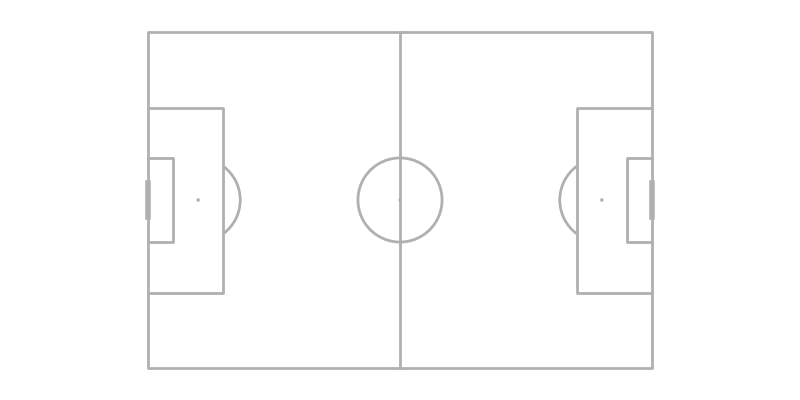## Draw on an existing axis

mplsoccer also plays nicely with other matplotlib figures. To draw a pitch on an existing matplotlib axis specify an `ax` in the `draw` method.

```fig, axs = plt.subplots(nrows=1, ncols=2)
pitch = Pitch()
pie = axs.pie(x=[5, 15])
pitch.draw(ax=axs)
```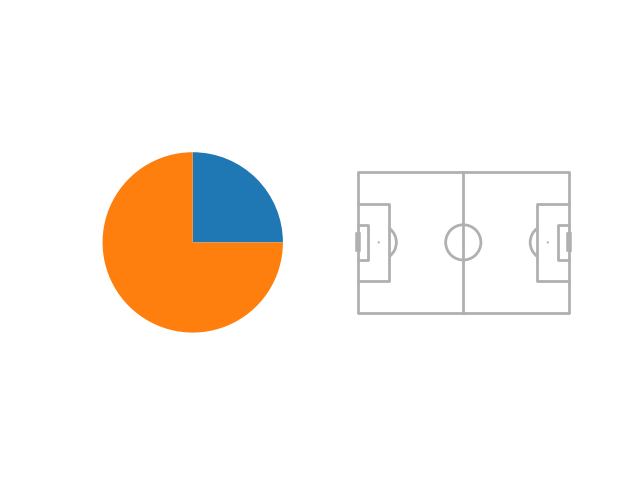## Supported data providers

mplsoccer supports 10 pitch types by specifying the `pitch_type` argument: ‘statsbomb’, ‘opta’, ‘tracab’, ‘wyscout’, ‘uefa’, ‘metricasports’, ‘custom’, ‘skillcorner’, ‘secondspectrum’ and ‘impect’. If you are using tracking data or the custom pitch (‘metricasports’, ‘tracab’, ‘skillcorner’, ‘secondspectrum’ or ‘custom’), you also need to specify the `pitch_length` and `pitch_width`, which are typically 105 and 68 respectively.

```pitch = Pitch(pitch_type='opta')  # example plotting an Opta/ Stats Perform pitch
fig, ax = pitch.draw()
```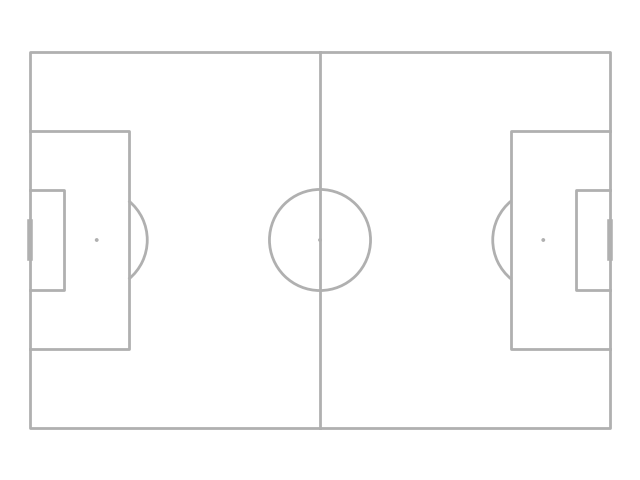```pitch = Pitch(pitch_type='tracab',  # example plotting a tracab pitch
pitch_length=105, pitch_width=68,
axis=True, label=True)  # showing axis labels is optional
fig, ax = pitch.draw()
```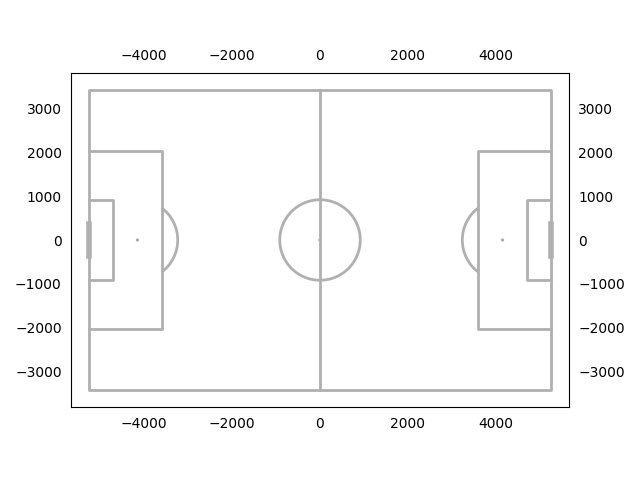mplsoccer also plots on grids by specifying nrows and ncols. The default is to use tight_layout. See: https://matplotlib.org/stable/tutorials/intermediate/tight_layout_guide.html.

```pitch = Pitch()
fig, axs = pitch.draw(nrows=2, ncols=3)
```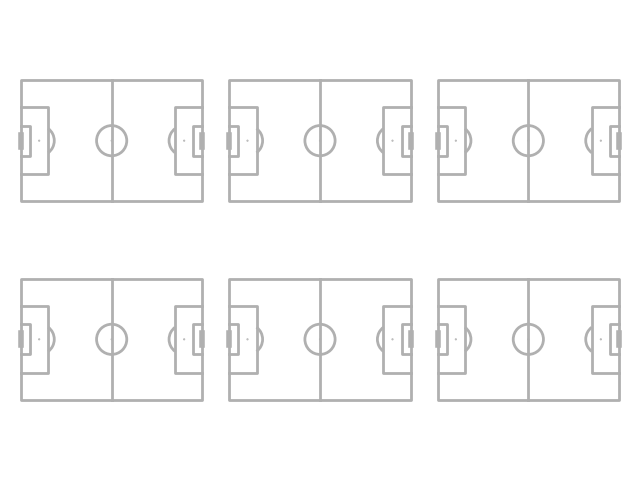But you can also use constrained layout by setting `constrained_layout=True` and `tight_layout=False`, which may look better. See: https://matplotlib.org/stable/tutorials/intermediate/constrainedlayout_guide.html.

```pitch = Pitch()
fig, axs = pitch.draw(nrows=2, ncols=3, tight_layout=False, constrained_layout=True)
```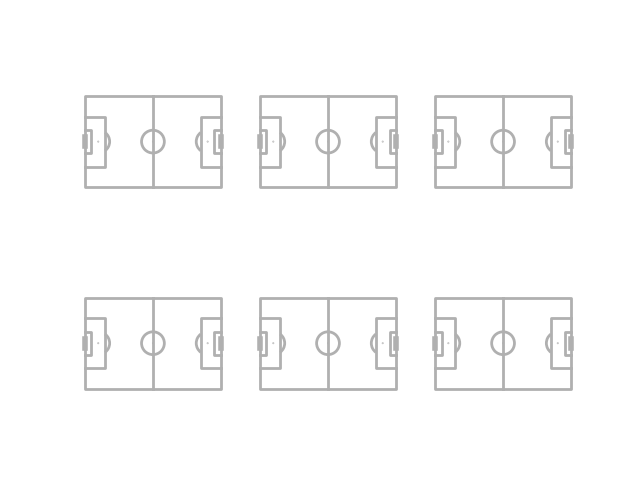If you want more control over how pitches are placed you can use the grid method. This also works for one pitch (nrows=1 and ncols=1). It also plots axes for an endnote and title (see the plot_grid example for more information).

```pitch = Pitch()
fig, axs = pitch.grid(nrows=3, ncols=3, figheight=10,
# the grid takes up 71.5% of the figure height
grid_height=0.715,
# 5% of grid_height is reserved for space between axes
space=0.05,
# centers the grid horizontally / vertically
left=None, bottom=None)
```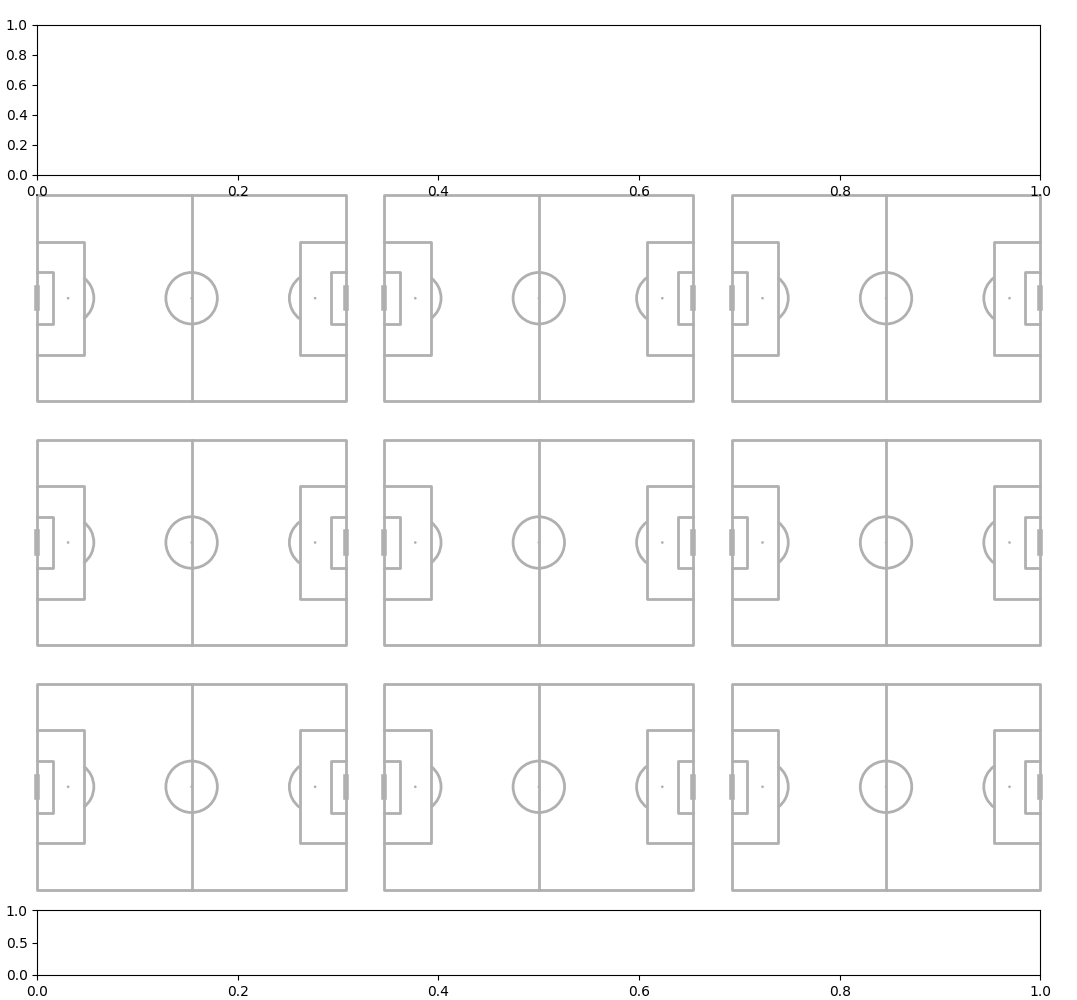## Pitch orientation

There are four basic pitch orientations. To get vertical pitches use the VerticalPitch class. To get half pitches use the half=True argument.

Horizontal full

```pitch = Pitch(half=False)
fig, ax = pitch.draw()
```Vertical full

```pitch = VerticalPitch(half=False)
fig, ax = pitch.draw()
```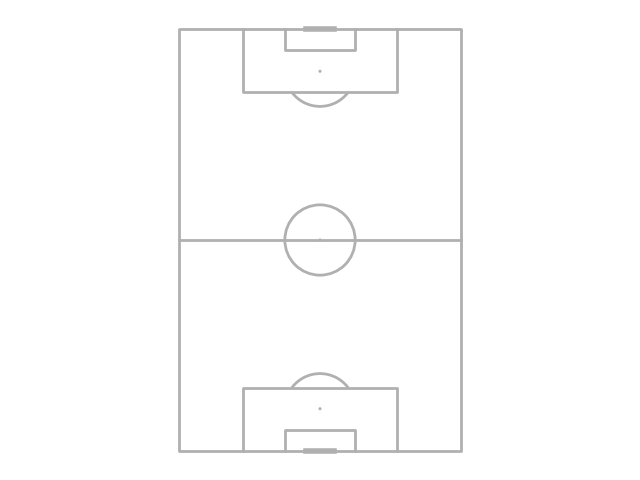Horizontal half

```pitch = Pitch(half=True)
fig, ax = pitch.draw()
```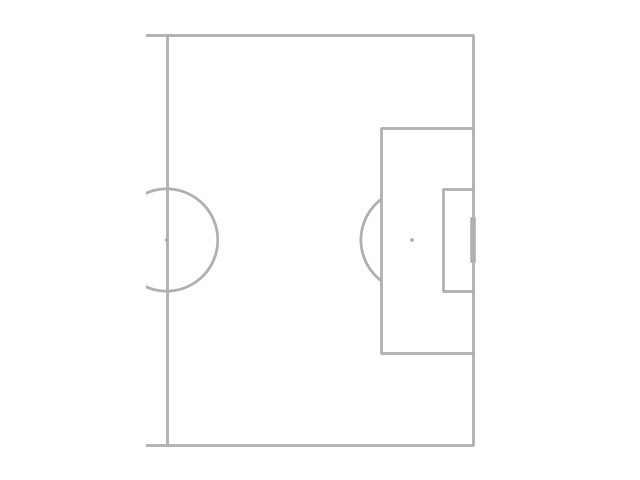Vertical half

```pitch = VerticalPitch(half=True)
fig, ax = pitch.draw()
```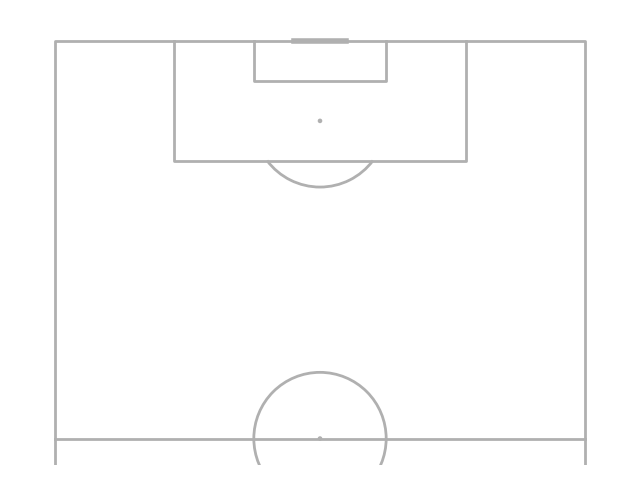You can also adjust the pitch orientations with the `pad_left`, `pad_right`, `pad_bottom` and `pad_top` arguments to make arbitrary pitch shapes.

```pitch = VerticalPitch(half=True,
pad_left=-10,  # bring the left axis in 10 data units (reduce the size)
pad_right=-10,  # bring the right axis in 10 data units (reduce the size)
pad_top=10,  # extend the top axis 10 data units
pad_bottom=20)  # extend the bottom axis 20 data units
fig, ax = pitch.draw()
```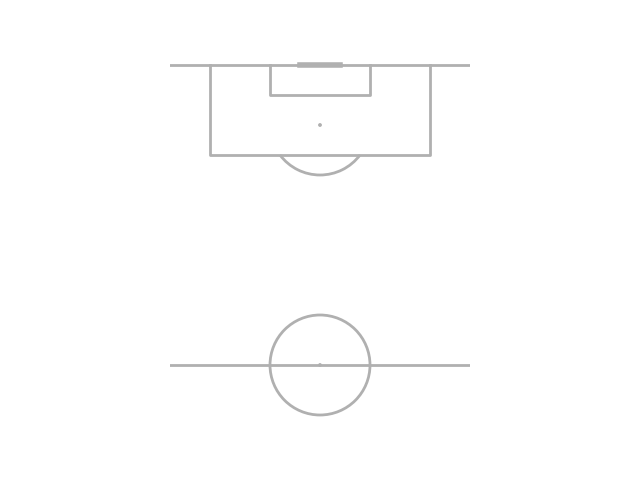## Pitch appearance

The pitch appearance is adjustable. Use `pitch_color` and `line_color`, and `stripe_color` (if `stripe=True`) to adjust the colors.

```pitch = Pitch(pitch_color='#aabb97', line_color='white',
stripe_color='#c2d59d', stripe=True)  # optional stripes
fig, ax = pitch.draw()
```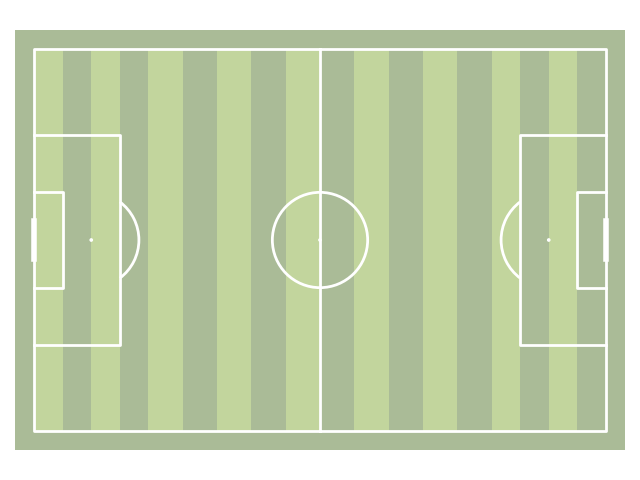## Line style

The pitch line style is adjustable. Use `linestyle` and `goal_linestyle` to adjust the colors.

```pitch = Pitch(linestyle='--', linewidth=1, goal_linestyle='-')
fig, ax = pitch.draw()
```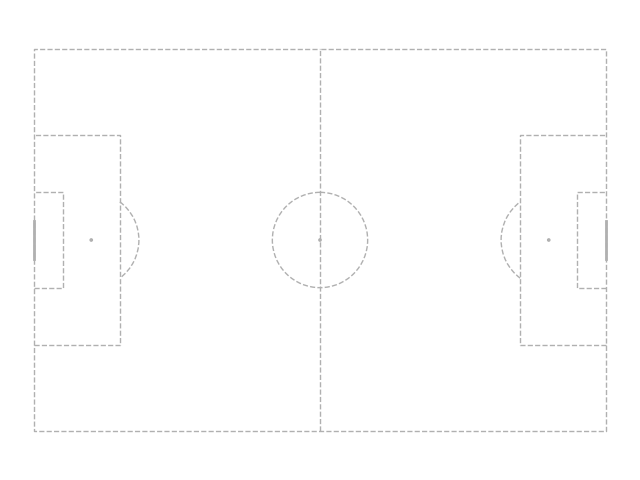## Line alpha

The pitch transparency is adjustable. Use `pitch_alpha` and `goal_alpha` to adjust the colors.

```pitch = Pitch(line_alpha=0.5, goal_alpha=0.3)
fig, ax = pitch.draw()
```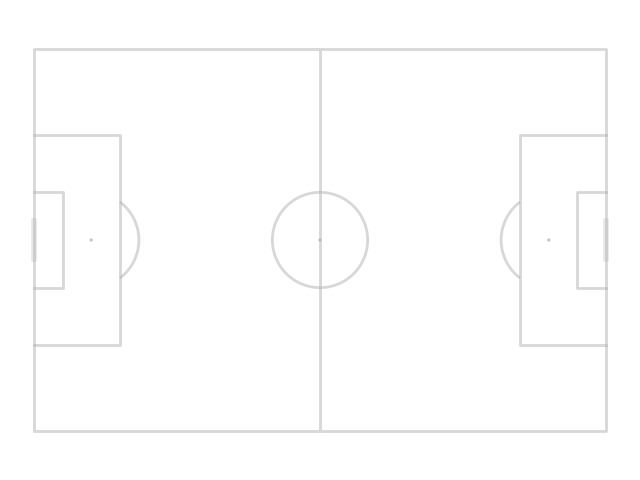## Corner arcs

You can add corner arcs to the pitch by setting `corner_arcs` = True

```pitch = VerticalPitch(corner_arcs=True, half=True)
fig, ax = pitch.draw(figsize=(10, 7.727))
```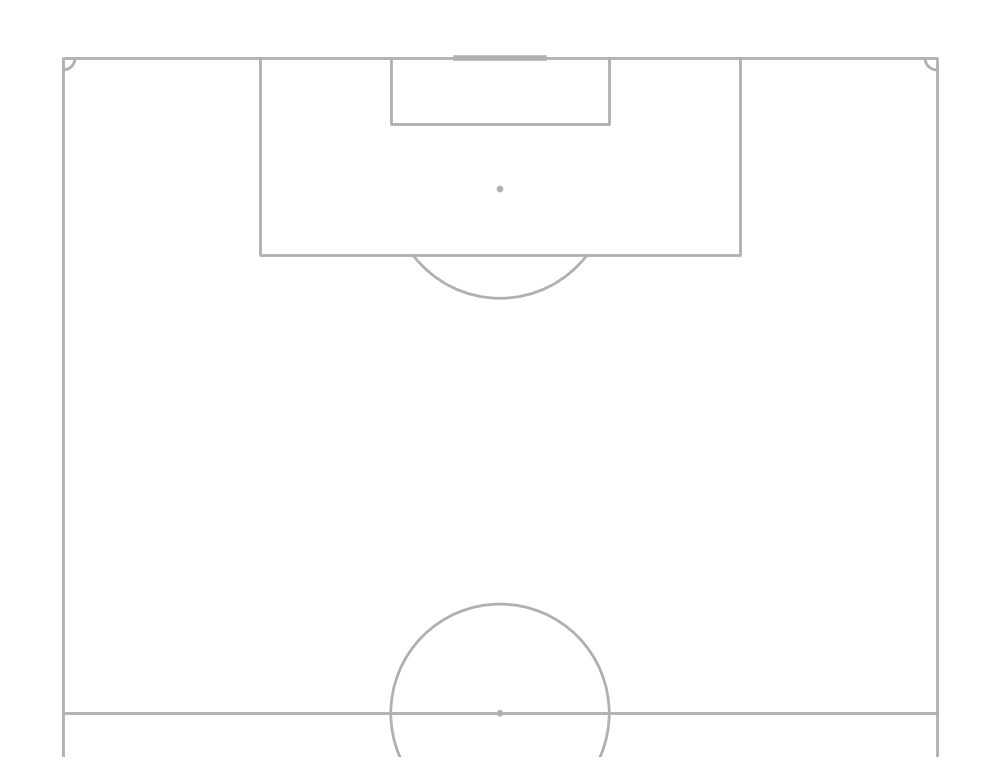## Juego de Posición

You can add the Juego de Posición pitch lines and shade the middle third. You can also adjust the transparency via `shade_alpha` and `positional_alpha`.

```pitch = Pitch(positional=True, shade_middle=True, positional_color='#eadddd', shade_color='#f2f2f2')
fig, ax = pitch.draw()
```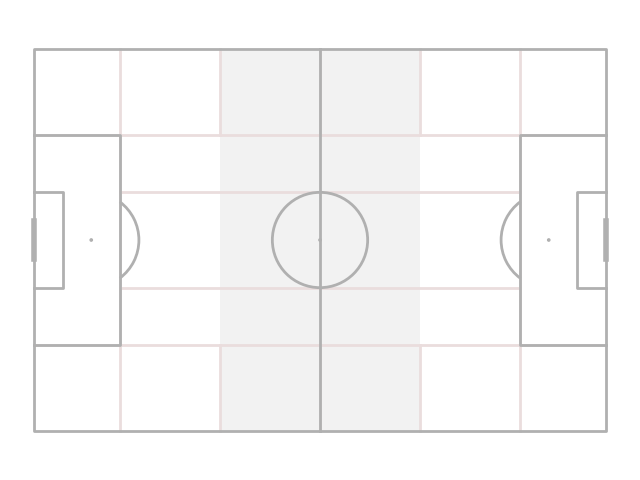mplsoccer can also plot grass pitches by setting `pitch_color='grass'`.

```pitch = Pitch(pitch_color='grass', line_color='white',
stripe=True)  # optional stripes
fig, ax = pitch.draw()
```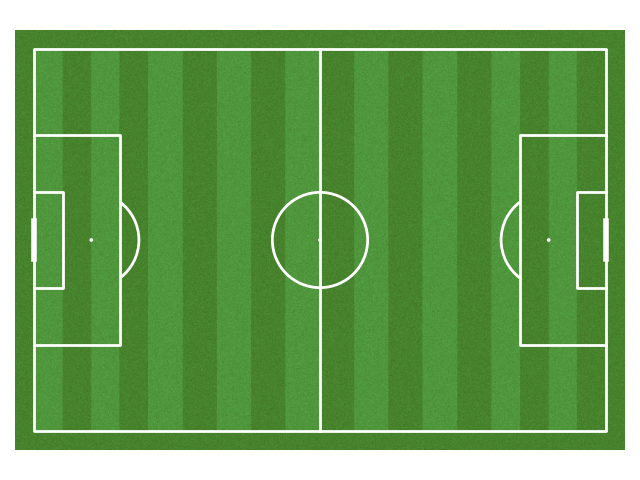Three goal types are included `goal_type='line'`, `goal_type='box'`, and `goal_type='circle'`

```fig, axs = plt.subplots(nrows=3, figsize=(10, 18))
pitch = Pitch(goal_type='box', goal_alpha=1)  # you can also adjust the transparency (alpha)
pitch.draw(axs)
pitch = Pitch(goal_type='line')
pitch.draw(axs)
pitch = Pitch(goal_type='circle', linewidth=1)
pitch.draw(axs)
```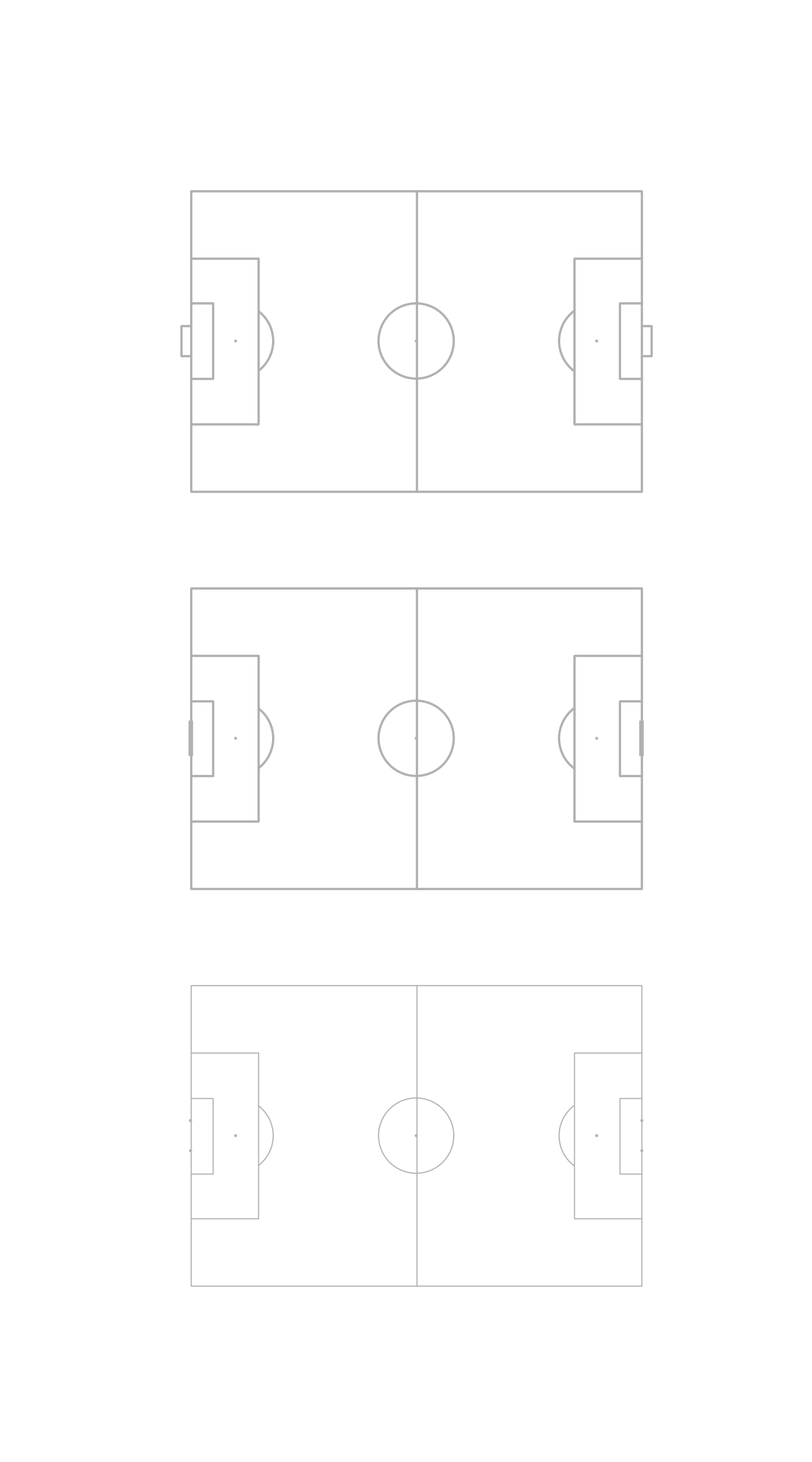The line markings and spot size can be adjusted via `linewidth` and `spot_scale`. Spot scale also adjusts the size of the circle goal posts.

```pitch = Pitch(linewidth=3,
# the size of the penalty and center spots relative to the pitch_length
spot_scale=0.01)
fig, ax = pitch.draw()
```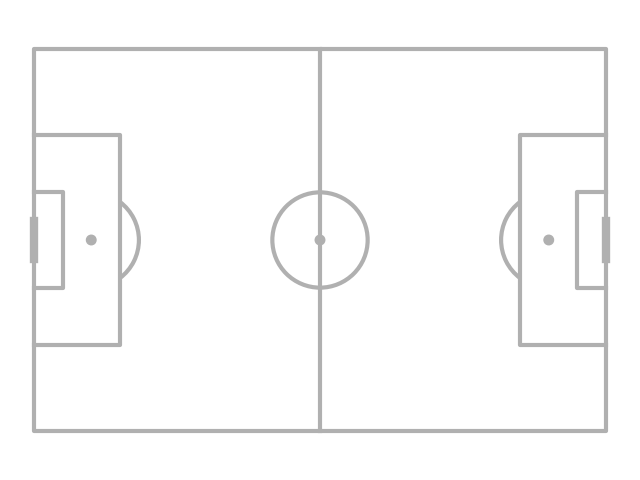If you need to lift the pitch markings above other elements of the chart. You can do this via `line_zorder`, `stripe_zorder`, `positional_zorder`, and `shade_zorder`.

```pitch = Pitch(line_zorder=2)  # e.g. useful if you want to plot pitch lines over heatmaps
fig, ax = pitch.draw()
```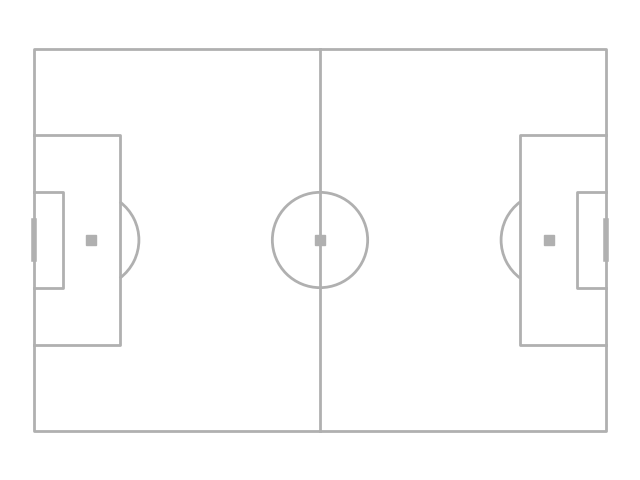## Axis

By default mplsoccer turns of the axis (border), ticks, and labels. You can use them by setting the `axis`, `label` and `tick` arguments.

```pitch = Pitch(axis=True, label=True, tick=True)
fig, ax = pitch.draw()
```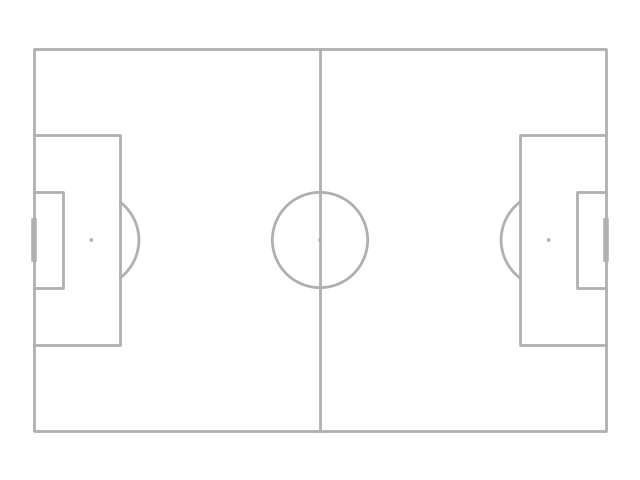## xkcd

Finally let’s use matplotlib’s xkcd theme.

```plt.xkcd()
pitch = Pitch(pitch_color='grass', stripe=True)
fig, ax = pitch.draw(figsize=(8, 4))
annotation = ax.annotate('Who can resist this?', (60, 10), fontsize=30, ha='center')

plt.show()  # If you are using a Jupyter notebook you do not need this line
```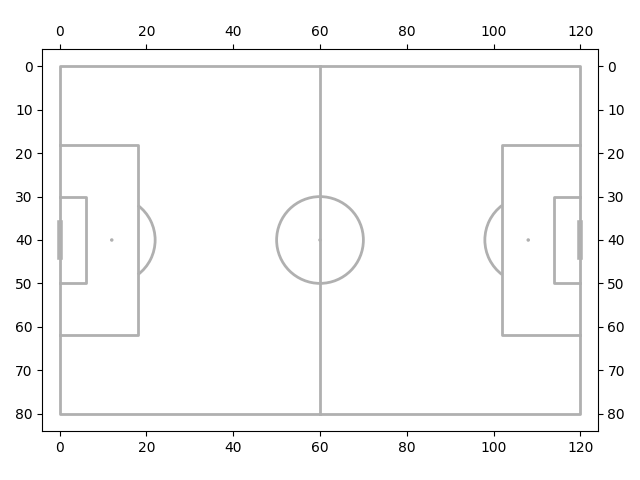Total running time of the script: (0 minutes 6.294 seconds)

Gallery generated by Sphinx-Gallery